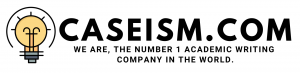# Portfolio Optimization Case Solution & Answer

## Portfolio Optimization Case Solution

Thus according to the net results, it is identified that the first period of 2006 was not good for the investment because it generated negative results under the given portfolio, the later period shows high returns as compared to the previous one. From the data, ten risk measures and expected returns have been calculated and related to each other in order to analyze which period shows best results.

After the period 3, the expected return increased by the particular rate and thus attracted the investorâ€™s attention to take decision about investing into the stock or to hold in order to receive high dividends. The ratio also shows that the final period did not generated the desire outcome due to the downfall of the market and to withdraw the shareholderâ€™s amount to minimize the risk factor.

Therefore, it is concluded that this expected result is the initial stage to allow the investor to make certain decisions about the future consideration within the company and the second thing they would consider is the level of risk under the expected return, which would hinder the results of the investorâ€™s criteria and decrease the shareholderâ€™s interest on retaining the stock.

Sharpe Ratio

The Sharpe ratio is another tool to assess the riskiness of the portfolio from the case, the negative Sharpe ratio represents the negative results of the portfolio and thus allows the investor to withdraw the amount to decrease the loss incurred under the investment.

The positive Sharpe ratio illustrates healthy portfolio in which the invested amount would generate the desired outcome and maintained for the long-term.The overall results show that the portfolio of the three companies indicate a healthy portfolio in which the investor would like to retain their investment for the long-term period in order to receive greater profits.

Portfolio optimization and conclusion

The portfolio optimization is the key tool to analyze the worth of a certain invested amount over the selected period of time. It also shows how much investment would be included in the near future.This means that if the portfolio optimization is positive,then it illustrates the addition to the certain invested amount.

So the net results shows that the portfolio would be attractive for the investorâ€™s criteria because it would generate positive portfolio optimization value, which would increase the amount of investment in the future. It is concluded that whether the risk factor is high within the stock, the return would always attract the investorâ€™s need to retain the stock or to sell under high value.

From the following results, it is analyzed that various sources would involve to generate the portfolio optimization value. The first thing to consider is the risk-free rate that would enable to reduce the risk factor, the second factor is the weights assigned to each firm under the portfolio. These weights are defined as the amount each weighted firm would consider when the portfolio would show desired rate. The third part of the optimization is the level of risk aversion that is the level of riskiness under the portfolio due to high standard deviation.

So to calculate the optimal portfolio value, the expected rate would be minimized by the risk-free rate and then divided by the risk aversion multiplied by 2 and the variance in order to generate the portfolio optimization. This value shows that if the portfolio would generate the value in positive percentage then it shows that the investment amount would increase by the selected proportion of the given under the calculated results………………..

This is just a sample partial work. Please place the order on the website to get your own originally done case solution